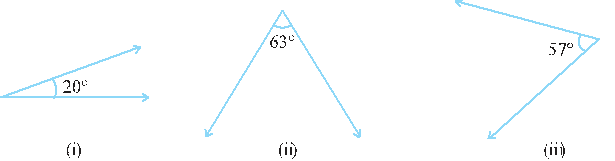# Ex.5.1 Q1 Lines and Angles Solution- NCERT Maths Class 7

Go back to  'Ex.5.1'

## Question

Find the complement of each of the followingVideo Solution
Lines & Angles
Ex 5.1 | Question 1

## Text Solution

What is known?

Measure of angles.

What is the unknown?

Measure of complementary angles.

Reasoning:

The sum of complementary angles is always If the given angle is $$x$$, then we can find complementary angle by subtracting from . Let us now find complement angles.

Steps:

i) Given angle $$=20^\circ$$

Complement angle of $$20^\circ$$

\begin{align} &= 90^{\circ} -\text{[given angle]} \\ &=90^{\circ}- 20^{\circ} \\ &=70^{\circ} \end{align}

(ii) Given angle  $$= 63^\circ$$

Complement angle of $$63^\circ$$

\begin{align} &= 90^{\circ} -\text{[given angle]} \\ &= 90^{\circ}-63^{\circ} \\ &= 27^{\circ} \end{align}

(iii) Given angle  $$= 57^\circ$$

Complement angle of $$57^\circ$$

\begin{align} &= 90^{\circ}- \text{[given angle]} \\ &= 90^{\circ}-57^{\circ} \\ &= 33^{\circ} \end{align}

Learn from the best math teachers and top your exams

• Live one on one classroom and doubt clearing
• Practice worksheets in and after class for conceptual clarity
• Personalized curriculum to keep up with school